# Quiz Discussion

Three taps A, B and C can fill a tank in 12,15 and 20 hours respectively. If A is open all the time and B and C are open for one hour each alternately, the tank will be full in.

Course Name: Quantitative Aptitude

• 1]

6 hours

• 2]

6 2/3 hours

• 3]

7 hours

• 4]

7 1/2 hours

##### Solution
No Solution Present Yet

#### Top 5 Similar Quiz - Based On AI&ML

Quiz Recommendation System API Link - https://fresherbell-quiz-api.herokuapp.com/fresherbell_quiz_api

# Quiz
1
Discuss

An outlet pipe can empty a cistern in 3 hours. In what time will empty 2/3 of the cistern?

• 1] 3 hours
• 2] 5 hours
• 3] 2 hours
• 4] 4 hours
##### Solution
2
Discuss

Two pipe can fill a cistern in 3 hours and 4 hours respectively and a waste pipe can empty it in 2 hours. If all the three pipes are kept open, then the cistern will be filled in-

• 1] 5 hours
• 2] 8 hours
• 3] 10 hours
• 4] 12 hours
##### Solution
3
Discuss

Pipes A and B can fill a tank in 5 and 6 hours respectively. Pipe C can empty it in 12 hours. If all the three pipes are opened together, then the tank will be filled in:

• 1]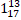hours

• 2]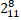hours

• 3]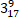hours

• 4]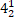hours

##### Solution
4
Discuss

Two pipes can fill a tank in 20 and 24 minutes respectively and a waste pipe can empty 3 gallons per minute. All the three pipes working together can fill the tank in 15 minutes. The capacity of the tank is:

• 1] 60 gallons
• 2] 100 gallons
• 3] 120 gallons
• 4] 180 gallons
• 5] None of these
##### Solution
5
Discuss

Two pipes A and B can fill a tank in 15 minutes and 20 minutes respectively. Both the pipes are opened together but after 4 minutes, pipe A is turned off. What is the total time required to fill the tank?

• 1]

10 min. 20 sec.

• 2]

11 min. 45 sec.

• 3]

12 min. 30 sec.

• 4]

14 min. 40 sec.

##### Solution
6
Discuss

Three pipes A, B and C can fill a tank from empty to full in 30 minutes, 20 minutes, and 10 minutes respectively. When the tank is empty, all the three pipes are opened. A, B and C discharge chemical solutions P, Q and R respectively. What is the proportion of the solution R in the liquid in the tank after 3 minutes?

• 1]

5/11

• 2]

6/11

• 3]

7/11

• 4]

8/11

##### Solution
7
Discuss

Two pipes A and B can fill a tank in 15 minutes and 20 minutes respectively. Both the pipes are opened together but after 4 minutes, pipe A is turned off. What is the total time required to fill the tank?

• 1] 10 min. 20 sec.
• 2] 11 min. 45 sec.
• 3] 12 min. 30 sec.
• 4] 14 min. 40 sec.
##### Solution
8
Discuss

Three pipes A, B and C can fill a tank in 6 hours. After working at it together for 2 hours, C is closed and A and B can fill the remaining part in 7 hours. The number of hours taken by C alone to fill the tank is:

• 1] 10
• 2] 12
• 3] 14
• 4] 16
##### Solution
9
Discuss

A pipe can fill a tank in x hours and another pipe can empty it in y (y > x) hours. If both pipes are open, in how many hours will the tank is filled?

• 1]

(x - y) hours

• 2]

(y - x) hours

• 3]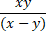hours

• 4]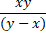hours

##### Solution
10
Discuss

Pipes A and B can fill a tank in 5 and 6 hours respectively. Pipe C can empty it in 12 hours. If all the three pipes are opened together, then the tank will be filled in:

• 1]

1 13/17 hours

• 2]

2 8/11 hours

• 3]

3 9/17 hours

• 4]

4 1/2 hours

# Quiz